# Magneto Static: non-meshed sources (3D)

## Introduction

The scalar model of Flux 3D allows sources of magnetic field of the non-meshed type (non meshed coils or magnetic field source).

## Definitions

Non-meshed coil:

A non-meshed coil is a geometric entity of circular, rectangular or more complex shape that is superimposed onto the mesh and has no dependence with it.

The computation of the magnetic field created by the current distribution is carried out using the Biot and Savart formula in an analytic way (or semi-analytic) as presented below.

Magnetic field source:

A magnetic field source is an external magnetic field (e.g. the earth's field) that is superimposed onto the internal magnetic field within the device.

Its value is imposed by the user.

## Non-meshed coil: principle of magnetic field computation

In the absence of the magnetic materials, the intensity of the magnetic field created in a point P of the space by a filiform conductor carrying a constant current I is computed with the formulas: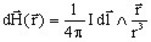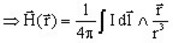where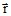is the vector between the element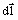of the conductor and the point where the magnetic field is computed.

For the volume or surface distributions of the current, the expression of the Biot and Savart formula must be rewritten. The term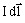becomes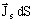for a surface distribution and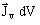for a volume distribution. Thus, the integrations are carried out on the surface of the surface conductor or on the volume of the solid conductor.

The analytic expression of the magnetic field intensity H is known for specific distributions of the currents (rectilinear wire, rectilinear sheet, circular turn…).

For complex current distributions, the computation is carried out after the separation into simpler elements.

## Non-meshed magnetic sources and the magnetic scalar potential

The magnetic field created by the non-meshed sources (non-meshed coils, or magnetic field sources) is written Hj. It corresponds to the Hj field, previously defined (see § Magnéto Static : solved equations (scalar model) )

In connection with the previous definitions:

• In the equation solved in magnetic reduced scalar potential with respect to Hj (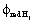) the sources are of the non-meshed type and the Hj field is created by these ones.
• In the equation solved in magnetic reduced scalar potential with respect to T0 (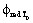) the sources can be of the meshed type and/or of the non-meshed type. The electric vector potential is written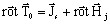, (where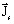is the current density in the meshed sources (conductors) and Hj is the field created by the non-meshed sources.